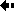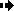## Rates of evolution - How do we measure the rate of evolution?The rate of evolution

The rate of evolution is a measurement of the change in an evolutionary lineage over time.

The method for measuring the rate of evolution can be illustrated by work done by MacFadden on horse teeth: horse teeth are classic materials in the study of evolution.

The rate of evolution is measured as follows:

Suppose that a character has been measured at two times, t1 and t2 ; t1 and t2 are expressed as times before the present in millions of years.

The time interval between the two samples can be written as:Dt = t1 - t2,

which is 1 million years if t1 = 15.2 and t2 =14.2 The average value of the character is defined as x1 in the earlier sample and x2 in the later sample; we then take natural logarithms of x1 and x2 (the natural logarithm is the log to base e where e = 2.718, and it is symbolized by ln ).

The evolutionary rate (r) then is

r = (ln(x2) - ln(x1)) / Dt

The rate of evolution is measured in 'darwins'.

Haldane (pictured opposite) defined a 'darwin' as a unit to measure evolutionary rates; one darwin is a change in the character by a factor of e in one million years.

The formula above for r gives the rate in darwins provided that the time interval is in millions of years.Previous Next